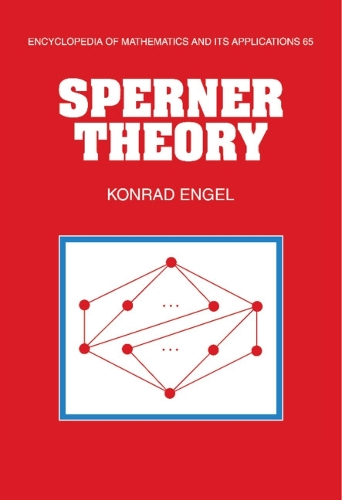•# Sperner Theory - Encyclopedia of Mathematics and its Applications (Hardback)

(author)
£92.00
Hardback 432 Pages / Published: 28/01/1997
• We can order this

Usually dispatched within 3 weeks

The starting point of this book is Sperner's theorem, which answers the question: What is the maximum possible size of a family of pairwise (with respect to inclusion) subsets of a finite set? This theorem stimulated the development of a fast growing theory dealing with external problems on finite sets and, more generally, on finite partially ordered sets. This book presents Sperner theory from a unified point of view, bringing combinatorial techniques together with methods from programming, linear algebra, Lie-algebra representations and eigenvalue methods, probability theory, and enumerative combinatorics. Researchers and graduate students in discrete mathematics, optimisation, algebra, probability theory, number theory, and geometry will find many powerful new methods arising from Sperner theory.

Publisher: Cambridge University Press
ISBN: 9780521452069
Number of pages: 432
Weight: 780 g
Dimensions: 234 x 156 x 24 mm